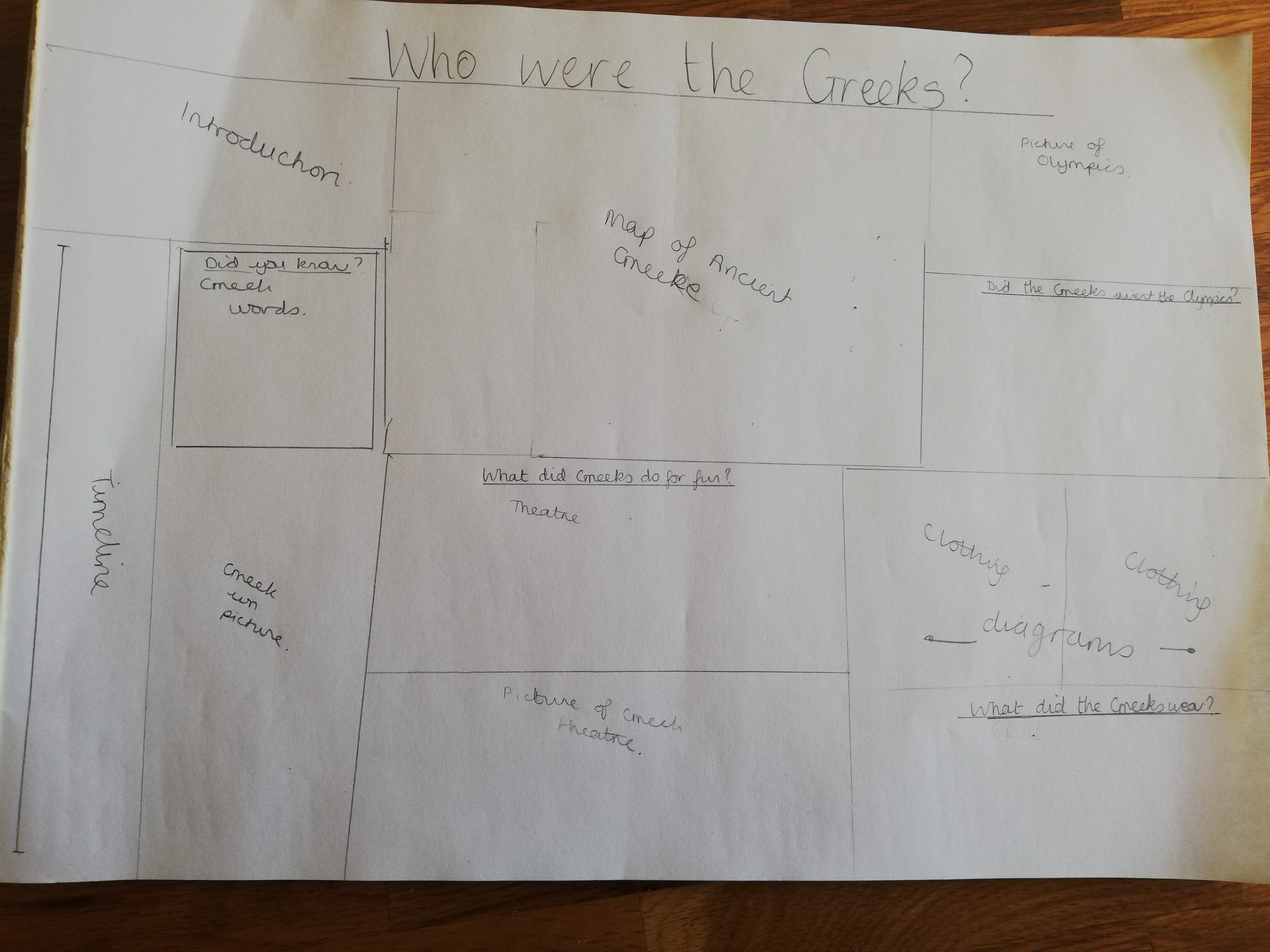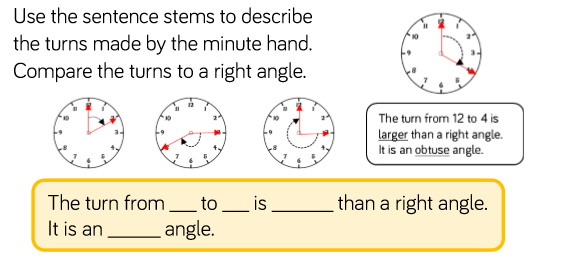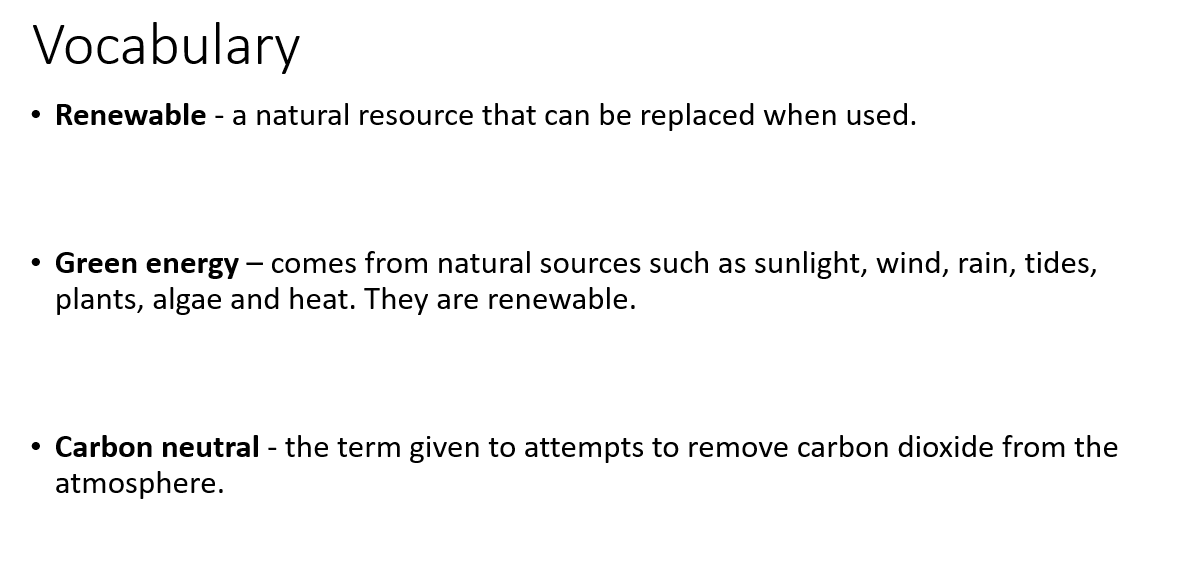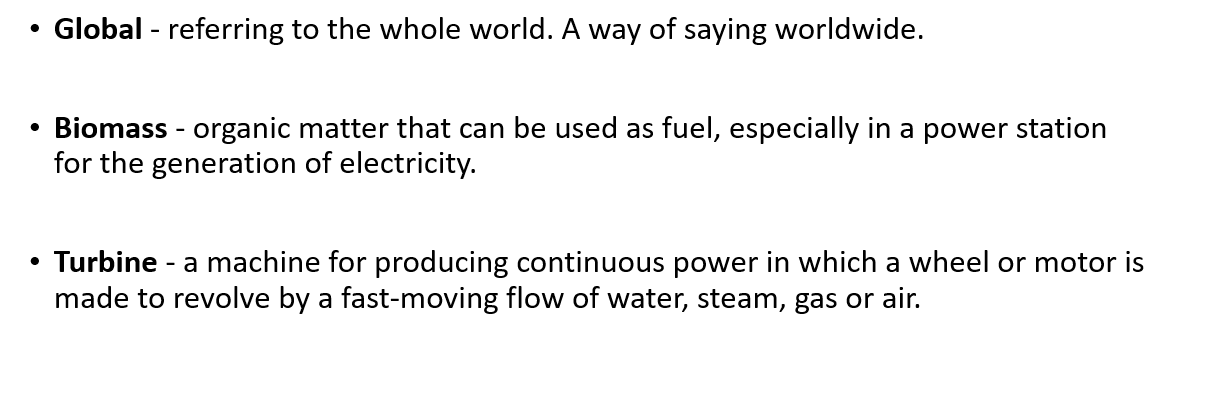# Monday 11th May – Year 5

Good morning Year 5. I hope you had a lovely weekend and possibly celebrated the 75th anniversary of VE Day.

### English

Plan your non-chronological report on the Greeks so that you are ready to write it up tomorrow. This doesn’t have to be put onto the blog but writing your plan on paper will help you immensely tomorrow. We always love getting your work via e mail too!

Here is a suggested version of how it might look. You don’t have to necessarily use this as you are also welcome to create your own design, but if you are stuck for ideas it’s a good starting point. Remember the example that you looked at last Thursday as this may also give you ideas.If you have been blogging and completing work regularly, you will already have all of the details that you need to go into each section. Remember that you looked at 5 important events, Olympics, government, clothing and theatre.

### Maths

Today we are going to start looking at angles. The following link should act as a bit of revision:

https://www.bbc.co.uk/bitesize/topics/zb6tyrd/articles/zg68k7h

1. Complete the following:
• Name and define the 4 types of angles
• What are angles measured in?
• What instrument is used for measuring angles?
• How many degrees in a half turn?
• How many degrees in a  full turn?

2.  Answer the following question. The right hand example tells you how to use the sentence stem to answer – then you need to use the same sentence stem to answer each of the three clock examples underneath.Now try some examples of your own.

Challenge – can you do an example that includes each of the 4 types of angles?

### GeographyWhat are renewable energy sources? How many can you list?

https://www.alliantenergykids.com/RenewableEnergy/RenewableEnergyHome

Choose one of the following and research it in more detail, blogging what you find.

• Solar energy
• Geothermal energy
• Hydroelectric energy
• Biomass energy
• Wind energy
• Tidal energy

Have a great day.

From Mrs Millard, Mrs Leeming and Mrs Munro XX

### 58 thoughts on “Monday 11th May – Year 5”

•11th May 2020 at 10:05 am

maths
1.right angle , reflex angle ,acute angle ,obtuse angle .
2.the angles are measured in degree (*).
3.an inclinometer is the instrument to measure angles .
4.180
5.360

•11th May 2020 at 11:12 am

1.
● Acute, Right, Obtuse and straight
● Degrees
● Protractor
● 180
● 360

2.
● The turn from 12 to 2 is smaller than a right angle so it is an acute angle
● The turn from 3 to 8 is larger than a right angle so it is an obtuse angle
● The turn from 12 to 3 is equal to a right angle.

My own:
● The turn from 4 to 10 is larger than a right angle so it is a straight angle.
● The turn from 9 to 11 is smaller than a right angle so it is an acute angle.

•11th May 2020 at 4:54 pm

Thanks Dan,
3) Angles are measured using a protractor.
Did you define the 4 types?

Mrs Millard XX

•11th May 2020 at 10:14 am

Maths
1. Reflex angle ,acute angle ,right angle , obtuse angle
2.degree angle
3. Measure angles is inclinometer
5.360,4.180

•11th May 2020 at 4:55 pm

Thanks Evie,

Angles are measured using a protractor. Did you define the 4 types?

Mrs Millard XX

•11th May 2020 at 10:14 am

I didn’t understand the second part of maths may I have some help.

•11th May 2020 at 4:55 pm

I’m not sure what you don’t understand Dan – tell me!

•11th May 2020 at 10:27 am

solar energy
hydro energy
wind energy
biomass energy
tidal energy

•11th May 2020 at 4:57 pm

Thanks Dan,

Did you choose one to research further?

Mrs Millard XX

•11th May 2020 at 10:27 am

Solar is the Latin word for sun and it’s a powerful source of energy. In fact the sun that shines on the earth in just one hour could meet world energy for an entire year ! We can use solar power in two different ways 1. Windmills and a heart source x see you tomorrow x🦋❤🌈🥰

•11th May 2020 at 4:57 pm

Thanks Evie,

Mrs Millard XX

•11th May 2020 at 11:11 am

Hi every one I’m liking this work it’s a bit hard miss

•11th May 2020 at 4:58 pm

Always trying to challenge you Leon!
Did you manage to do any of it?

Mrs Millard XX

•11th May 2020 at 11:26 am

Geography
Wind energy:

Wind energy is powered by turbines that are normaly in big countrysides or in the sea the turbines are electrical ,wind will turn the turbines which spins a generator and makes electricity.But it doesnt make much energy but atleast is clean.

•11th May 2020 at 4:59 pm

Thanks Mason,

Do you know any other renewable energy sources?

Mrs Millard XX

•11th May 2020 at 11:31 am

They like theatre
They make people happy
The would which people dong a show
The Greek save are life
They were scaths what are cloths and the scaths Luke like cloths they were 👹👹👹🤡🤡😅😅

•11th May 2020 at 12:19 pm

4 angles
Right angle = 90°
Acute angle =less than 90°
Obtuse angle = more than 90°
Straight angle =180°
Angle are measured in degrees
Protractor
180°
360°
The turn from 12 to 2 is smaller than a right angle it is a acute angle.
The turn from 3 to 8 is bigger than a right angle itis a obtuse angle
The turn from 12to3 is the same as a right angle it is a right angle
The turn 3to6 issmaller than a right angle it is a acute angle
The turn 12to6 is bigger than a right angle is is a straight angle
The turn 4to9 is bigger than a right angle it is a obtuse angle
The turn 9to12 is the same as a right angle it is a right angle

•11th May 2020 at 5:08 pm

Hi Logan,

You’re right – an obtuse angle is more than 90 degrees. What’s it smaller than too?

Check the turn from 3 to 6 – is it acute?

Mrs Millard XX

•11th May 2020 at 5:00 pm

Thanks Leon,

How did the Greeks save our lives?

Mrs Millard XX

•11th May 2020 at 11:32 am

Maths

1.Right angle ,Acute angle, Obtuse angle,Reflex angle.
2.Angles are measured in degrees.
3.Protractor.
4. 180
5. 360

•11th May 2020 at 5:01 pm

Did you define the 4 types?
Did you manage the second part?

Mrs Millard XX

•11th May 2020 at 5:31 pm

i didn’t get it so i didn’t do it.

•11th May 2020 at 5:31 pm

Maybe try it tomorrow??

•11th May 2020 at 11:41 am

Maths

The right angle is an angle at 90°.

An obtuse angle is it angle in between 90° to 180°.

An acute angle is an angle between 90° to 0°.

A rewind angle is an angle that is left from another angle.

•11th May 2020 at 5:02 pm

Thanks Simar,

What’s a reflex angle?

Mrs Millard Xx

•12th May 2020 at 11:17 am

Sorry
I had called a reflex angle a rewind angle

•11th May 2020 at 11:45 am

Maths

Angles are measured in degrees
Do you use a protractor to measure an angle
A half turn is 180°
A full turn is 360°

•11th May 2020 at 5:03 pm

Mrs Millard XX

•11th May 2020 at 11:50 am

geography
Solar energy.
Wind energy.
Hydro energy.
Tidal energy.
Geothermal energy.
Biomass energy.

Solar energy is the most abundant, renewable energy source in the world.
It’s systems refer to technologies that convert the sun’s heat or light to another form of energy for use.There are two categories of technologies that harness solar energy, Solar Photovoltaics and Solar Thermal.Solar Photovoltaic is a technology that converts sunlight into direct current electricity by using semiconductors. In contrast, Solar Thermal is a technology that utilizes the heat energy from the sun for heating or electricity production.

•11th May 2020 at 5:04 pm

Very well answered – well done!
What’s a ‘semiconductor’? Make sure that you don’t just copy details straight from the internet.

Mrs Millard XX

•11th May 2020 at 5:29 pm

ok miss i wont next time

•11th May 2020 at 5:33 pm

Well done – you never learn so well this way. It’s always better to put it into your own words that you understand!!

Make sure that the word ‘I’ is a capital letter!

•11th May 2020 at 11:53 am

Maths

The turn from 12 till two it’s less than a right angle it is an acute angle

The Turn from 3 to 8 is more than a right angle it’s an obtuse angle
The turn from 12 till three is the same as the right angle

•11th May 2020 at 5:09 pm

Thanks Simar,

Did you include some examples of your own?

Mrs Millard XX

•11th May 2020 at 12:54 pm

MATHS

Reflex angle, right angle, obtuse angle and an acute angle.

Right angle : 90 degrees
Obtuse: 90 -180 degrees
Acute : 90 – 0 degrees
Reflex: more than 180 degrees

Inclometre

360 and 180

•11th May 2020 at 5:10 pm

Thanks Dhanshri,

Angles are measured using a protractor.

Mrs Millard XX

•12th May 2020 at 1:43 pm

Ok mrs

•11th May 2020 at 1:16 pm

Geography

Geothermal energy is made from heat and steam spinning a turbine and generating electricity. Geothermal energy is pollution free , renewable and consistently reliable. This heat can come from volcanoes and geysers. 🔥💥💥

•11th May 2020 at 5:11 pm

Thanks Simar,

What other types of renewable energy sources do you know?

Mrs Millard XX

•11th May 2020 at 1:21 pm

The turn from 12 to 2 is less then a right angle so it’s an acute angle.
The turn from 12 to 3 is more than an acute angle so it’s a right angle.
The turn from 3 to 8 is more than an obtuse angle so it’s a reflex angle.

•11th May 2020 at 5:12 pm

Thanks Dhanshri,

Did you also include some examples of your own?

Mrs Millard XX

•11th May 2020 at 1:32 pm

Maths
1. The four main types of angles is right angles , acute angles, obtuse angles and straight angles.
2. An angle measure can be defined as the measure of the angle formed by the two rays or arms at a common vertex.
3. If you want to measure a angle you can use a protractor.

•11th May 2020 at 5:14 pm

Thanks Alex,

Do you know what a reflex angle is?
How many degrees are in a half and full turn?
An angle is a measurement of turn between two points. Do you fully understand what you wrote for 2)?

Mrs Millard XX

•11th May 2020 at 1:43 pm

Hydroelectric energy is made by flowing water that is captured and turned into electricity. When water from a damn is released it has to go through a turbine to produce electricity.

•11th May 2020 at 5:15 pm

Thanks Dhanshri,

Do you know any other types of renewable energy sources?

Mrs Millard XX

•11th May 2020 at 2:00 pm

Right angle,Obtuse,Acute,Reflex
Angles are measured in degrees
A protractor
A half turn is 180 degrees
A full turn is 360 degrees

•11th May 2020 at 5:16 pm

Did you define the 4 types?

Mrs Millard XX

•11th May 2020 at 2:07 pm

The turn from 12 to 2 is smaller than a right angle it is an acute angle.
The turn from 3 to 8 is bigger than a right angle it is an obtuse angle
The turn from 12 to 3 is equal to a right angle it is a right angle

•11th May 2020 at 2:14 pm

Solar energy

Solar energy is radiant light and heat from the sun which is harnessed using a range of every evolving technologies such as solar heating photovoltaic solar thermal energy solar architecture molten salt power plants and artificial photosynthesis

•11th May 2020 at 5:17 pm

Thanks Aamirat,

What does ‘harnessed’ and ‘photovoltaic’ mean?
Don’t forget full stops!

Do you know any other types of renewable energy sources?

Mrs Millard XX

•11th May 2020 at 3:11 pm

The missed maths
2. Angles are measured in degrees.
4. In a half turn is 180 degrees.
5. In a full turn is 360 degrees.
Maths 2.
The turns from 12 to 2 is smaller than right angle. It is an acute angle.
The turns from 3 to 8 is larger than a right angle. It is an obtuse angle.
The turns from 12 to 3 is the same than right angle .It is a straight angle.
The turns from 12 to 4 is bigger than a acute angle .It is a right angle.
The turns from 12 to 2 is smaller than obtuse .it is a acute angle.

•11th May 2020 at 5:19 pm

Well done Alex.

Do you know what acute, right angled and obtuse angles mean?

The turn from 12 to 3 is a right angle but is it also a straight line?
The turn from 12 to 4 is an obtuse angle – it’s bigger than a right angle.

Mrs Millard XX

•11th May 2020 at 3:51 pm

Geography
Renewable energy is made from resources that nature will replace, like wind, water and sunshine .
Wind energy can be called turbine witch gets electric from wind. they will be found in farms or wind farms. Wind farms have allot of turbines so have lots of energy .
See you tomorrow.

•11th May 2020 at 5:19 pm

Thanks Alex!

•11th May 2020 at 4:44 pm

Maths:
Acute angle 90 degrees and < 180 degrees
Straight angle is exactly 180 degrees
Angles are measured in degrees
Protractor
180 degrees is a half turn
360 degrees is a full turn.

Maths 2:
The turn from 12 to 2 is less than a right angle. It is an acute angle
The turn from 3 to 8 is more than a right angle. It is an obtuse angle
The turn from 3 to 12 is more than a right angle. It is an obtuse angle

•11th May 2020 at 5:21 pm

Thanks Zain,

An acute angle is less than 90 degrees.
Do you know what these are – obtuse, right angled and reflex angles?

The turn from 3 to 12 is a reflex angle.

Mrs Millard XX

•11th May 2020 at 4:57 pm

Geography:

Sunlight, wind, rain, tides, plants, algae and heat are renewable.

Biomass energy in the use of organic material to generate energy.Biomass is just organic matter like plants, wood pelletts and grass clippings even poop.

•